### Practice Set 6.6 Statistics Class 10th Mathematics Part 1 MHB Solution

Practice Set 6.6
1. The age group and number of persons, who donated blood in a blood donation camp is…
2. The marks obtained by a student in different subjects are shown. Draw a pie diagram…
3. In a tree plantation programme, the number of trees planted by students of different…
4. The following table shows the percentages of demands for different fruits registered…
5. The pie diagram in figure 6.13 shows the proportions of different workers in a town.…
6. The annual investments of a family are shown in the adjacent pie diagram. Answer the…
###### Practice Set 6.6
Question 1.

The age group and number of persons, who donated blood in a blood donation camp is given below. Draw a pie diagram from it.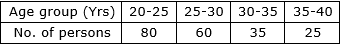Let us find the measures of central angles and show them in a table.

Know that,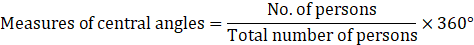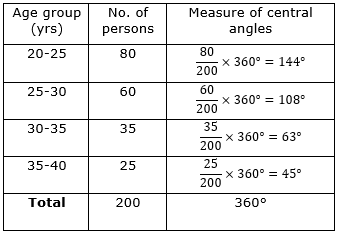Now we shall show the table into a pie chart.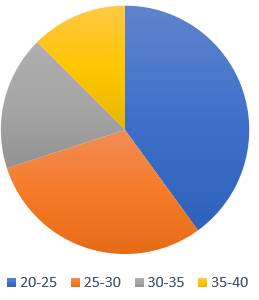Question 2.

The marks obtained by a student in different subjects are shown. Draw a pie diagram showing the information.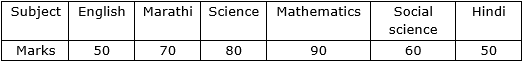Let us find the measures of central angles and show them in a table.

Know that,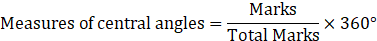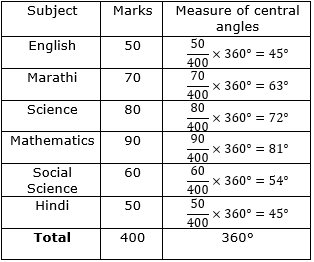Now we shall show the table into a pie chart.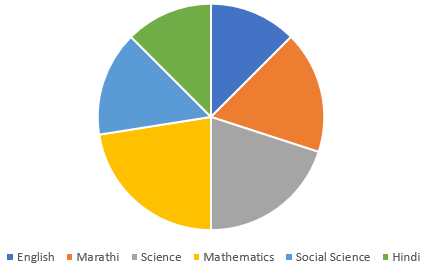Question 3.

In a tree plantation programme, the number of trees planted by students of different classes is given in the following table. Draw a pie diagram showing the information.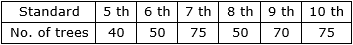Let us find the measures of central angles and show them in a table.

Know that,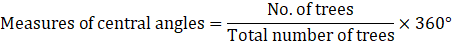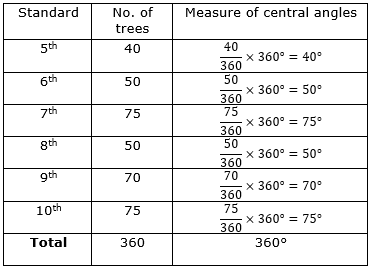Now we shall show the table into a pie chart.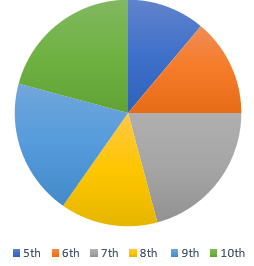Question 4.

The following table shows the percentages of demands for different fruits registered with a fruit vendor. Show the information by a pie diagram.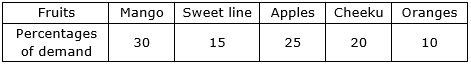Let us find the measures of central angles and show them in a table.

Know that,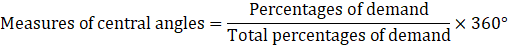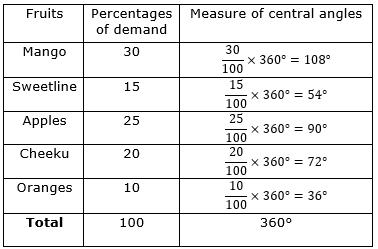Now we shall show the table into a pie chart.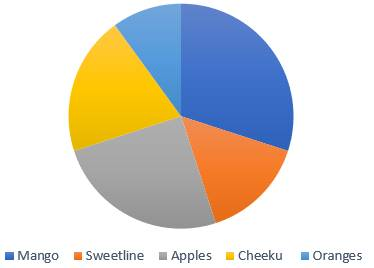Question 5.

The pie diagram in figure 6.13 shows the proportions of different workers in a town.

Answer the following questions with its help.

(1) If the total workers is 10,000; how many of them are in the field of construction?

(2) How many workers are working in the administration?

(3) What is the percentage of workers in production?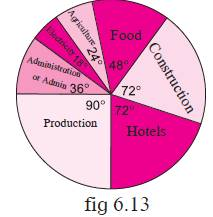(1) Given: total number of workers = 10000

And central angle for number of workers in construction = 72°

And we know that,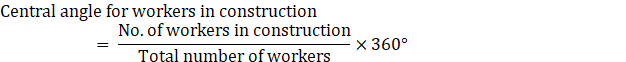⇒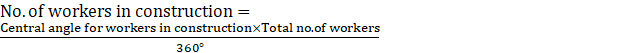⇒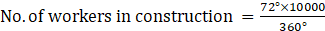⇒ No. of workers in construction = 2000

Thus, number of workers in the field of construction = 2000.

(2) We know that,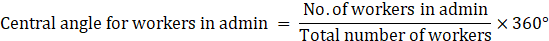⇒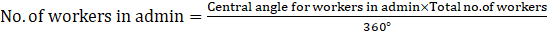⇒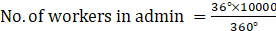⇒ No. of workers in admin = 1000

Thus, number of workers working in administration = 1000

(3) First, let us find the number of workers working in production.

We know that,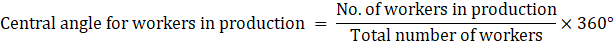⇒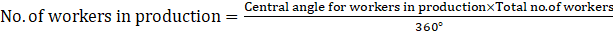⇒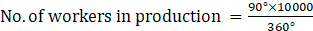⇒ No. of workers in production = 2500

In terms of percentage,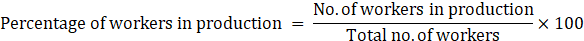⇒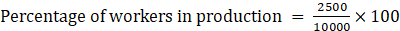⇒ Percentage of workers in production = 25%

Thus, percentage of workers in production = 25%

Question 6.

The annual investments of a family are shown in the adjacent pie diagram. Answer the following questions based on it.

(1) If the investment in shares is Rs 2000/, find the total investment.

(2) How much amount is deposited in bank?

(3) How much more money is invested in immovable property than in mutual fund?

(4) How much amount is invested in post?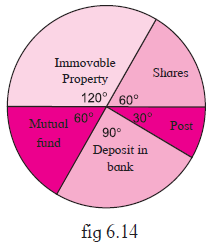(1) Given: Investment in shares = Rs. 2000

And central angle for investment in shares = 60°

And we know that,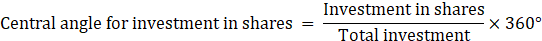⇒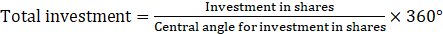⇒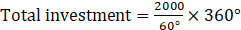⇒ Total investment = 12000

Thus, total investment is Rs. 12000.

(2) We know that, total investment = Rs. 12000

Central angle for deposits in banks = 90°

And we know that,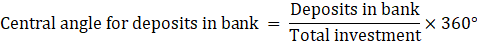⇒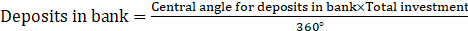⇒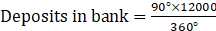⇒ Deposits in bank = 3000

Thus, deposits in bank is Rs. 3000.

(3) Firstly, let us find money invested in immovable property.

We know that, total investment = Rs. 12000

Central angle for investment in immovable property = 120°

And we know that,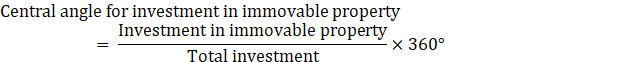⇒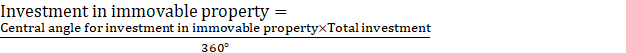⇒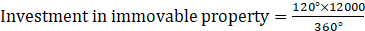⇒ Investment in immovable property = 4000 …(i)

Now, let us find money invested in mutual fund.

We know that, total investment = Rs. 12000

Central angle for investment in mutual fund = 60°

And we know that,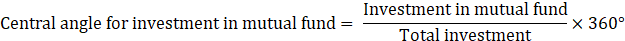⇒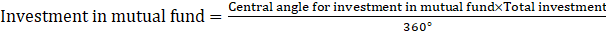⇒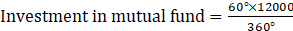⇒ Investment in mutual fund = 2000 …(ii)

Subtract (ii) from (i),

The additional money invested in immovable property than mutual fund = (Investment in immovable property) – (Investment in mutual fund)

= 4000 – 2000

= 2000

Thus, Rs. 2000 more money is invested in immovable property than mutual fund.

(4) We know that, total investment = Rs. 12000

Central angle for investment in post = 30°

And we know that,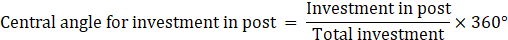⇒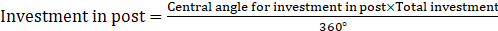⇒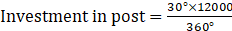⇒ Investment in post = 1000

Thus, investment in post is Rs. 1000.

## PDF FILE TO YOUR EMAIL IMMEDIATELY PURCHASE NOTES & PAPER SOLUTION. @ Rs. 50/- each (GST extra)

SUBJECTS

HINDI ENTIRE PAPER SOLUTION

MARATHI PAPER SOLUTION
SSC MATHS I PAPER SOLUTION
SSC MATHS II PAPER SOLUTION
SSC SCIENCE I PAPER SOLUTION
SSC SCIENCE II PAPER SOLUTION
SSC ENGLISH PAPER SOLUTION
SSC & HSC ENGLISH WRITING SKILL
HSC ACCOUNTS NOTES
HSC OCM NOTES
HSC ECONOMICS NOTES
HSC SECRETARIAL PRACTICE NOTES

2019 Board Paper Solution

HSC ENGLISH SET A 2019 21st February, 2019

HSC ENGLISH SET B 2019 21st February, 2019

HSC ENGLISH SET C 2019 21st February, 2019

HSC ENGLISH SET D 2019 21st February, 2019

SECRETARIAL PRACTICE (S.P) 2019 25th February, 2019

HSC XII PHYSICS 2019 25th February, 2019

CHEMISTRY XII HSC SOLUTION 27th, February, 2019

OCM PAPER SOLUTION 2019 27th, February, 2019

HSC MATHS PAPER SOLUTION COMMERCE, 2nd March, 2019

HSC MATHS PAPER SOLUTION SCIENCE 2nd, March, 2019

SSC ENGLISH STD 10 5TH MARCH, 2019.

HSC XII ACCOUNTS 2019 6th March, 2019

HSC XII BIOLOGY 2019 6TH March, 2019

HSC XII ECONOMICS 9Th March 2019

SSC Maths I March 2019 Solution 10th Standard11th, March, 2019

SSC MATHS II MARCH 2019 SOLUTION 10TH STD.13th March, 2019

SSC SCIENCE I MARCH 2019 SOLUTION 10TH STD. 15th March, 2019.

SSC SCIENCE II MARCH 2019 SOLUTION 10TH STD. 18th March, 2019.

SSC SOCIAL SCIENCE I MARCH 2019 SOLUTION20th March, 2019

SSC SOCIAL SCIENCE II MARCH 2019 SOLUTION, 22nd March, 2019

XII CBSE - BOARD - MARCH - 2019 ENGLISH - QP + SOLUTIONS, 2nd March, 2019

HSC Maharashtra Board Papers 2020

(Std 12th English Medium)

HSC ECONOMICS MARCH 2020

HSC OCM MARCH 2020

HSC ACCOUNTS MARCH 2020

HSC S.P. MARCH 2020

HSC ENGLISH MARCH 2020

HSC HINDI MARCH 2020

HSC MARATHI MARCH 2020

HSC MATHS MARCH 2020

SSC Maharashtra Board Papers 2020

(Std 10th English Medium)

English MARCH 2020

HindI MARCH 2020

Hindi (Composite) MARCH 2020

Marathi MARCH 2020

Mathematics (Paper 1) MARCH 2020

Mathematics (Paper 2) MARCH 2020

Sanskrit MARCH 2020

Important-formula

THANKS